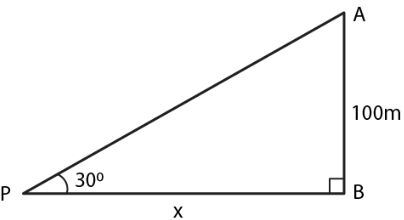Guru

# From a point P on level ground, the angle of elevation of the top of a tower is 300. If the tower is 100 m high, how far is P from the foot of the tower?

• 0

sir this is the question from the book -ML aggarwal( avichal publication) class 10th , chapter20 , heights and distances
we have the information that From a point P on level ground,
the angle of elevation of the top of a tower is 300. If the tower is 100 m high,
now we have to find how far is P from the foot of the tower.

question no 6 , heights and distances , ICSE board, ML Aggarwal

Share

1. Consider AB as the tower and P is at a distance of x m from B which is the foot of the tower.

Height of the tower = 100 m

Angle of elevation = 300We know that

tan θ = AB/PB

Substituting the values

tan 300 = 100/x

So we get

1/√3 = 100/x

By cross multiplication

x = 100√3

x = 100 (1.732) = 173.2 m

Hence, the distance of P from the foot of the tower is 173.2 m.

• 0